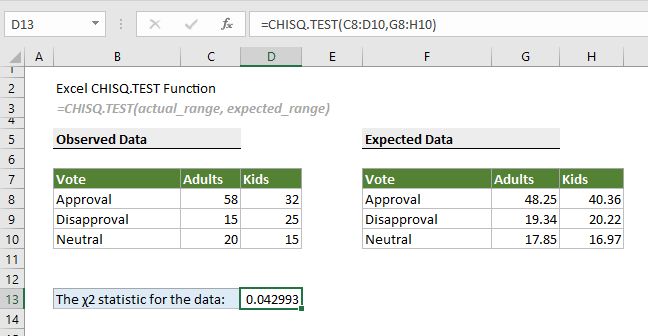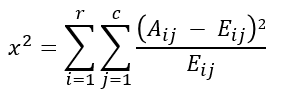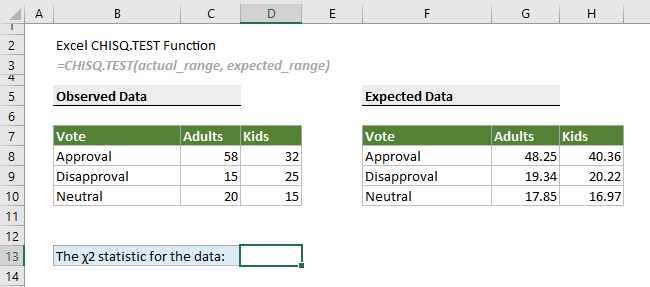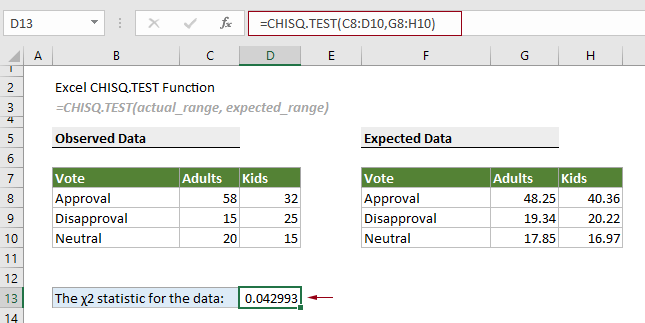## Excel CHISQ.TEST function

The CHISQ.TEST function calculates the chi-squared distribution of two provided data sets (the observed and expected frequencies), which can be used to test the independence of two categorical variables.Note: This CHISQ.TEST function is only available in Excel 2010 and later versions.

#### Syntax

=CHISQ.TEST(actual_range, expected_range)

#### Arguments

Actual_range (required): An array of observed frequencies used to test against the expected data.
Expected_range (required): An array of expected frequencies.

#### Remarks

1. The #N/A error occurs if either:
-- Two supplied arrays have different numbers of cells;
-- Either of the two arrays contains only one cell.
2. The #NUM! error occurs when any of the values in the expected data range is negative;
3. The #DIV/0! error occurs when any of the values in the expected data range is zero;
4. The equation of the CHISQ.TEST function is as follows.where,
Aij is the actual or observed frequencies in the i’th row and j’th column;
Eij is the expected frequencies in the i’th row and j’th column;
r is the number of rows;
c is the number of columns.

#### Return value

It returns a numeric value.

#### Example

To calculate the chi-squared distribution of two provided data sets (the observed and expected data in the screenshot below), you can do as follows.Select a cell to output the result, for example D13, enter the formula below and press the Enter key.

=CHISQ.TEST(C8:D10,G8:H10)#### Related Functions

Excel CHISQ.INV function
The CHISQ.INV function calculates the inverse of the left-tailed probability of the chi-squared distribution.

Excel CHISQ.INV.RT function
The CHISQ.INV.RT function calculates the inverse of the right-tailed probability of the chi-squared distribution.

Excel CONFIDENCE.NORM function
The CONFIDENCE.NORM function uses a normal distribution to calculate the confidence interval for a population mean.

Excel CONFIDENCE.T function
The CONFIDENCE.T function uses a student’s distribution to calculate the confidence interval for a population mean.

### The Best Office Productivity Tools

#### Kutools for Excel - Helps You To Stand Out From Crowd

 Popular Features: Find, Highlight or Identify Duplicates  |  Delete Blank Rows  |  Combine Columns or Cells without Losing Data  |  Round without Formula ... Super VLookup: Multiple Criteria  |  Multiple Value  |  Across Multi-Sheets  |  Fuzzy Lookup... Adv. Drop-down List: Easy Drop Down List  |  Dependent Drop Down List  |  Multi-select Drop Down List... Column Manager: Add a Specific Number of Columns  |  Move Columns  |  Toggle Visibility Status of Hidden Columns  |  Compare Columns to Select Same & Different Cells ... Featured Features: Grid Focus  |  Design View  |  Big Formula Bar  |  Workbook & Sheet Manager | Resource Library (Auto Text)  |  Date Picker  |  Combine Worksheets  |  Encrypt/Decrypt Cells  |  Send Emails by List  |  Super Filter  |  Special Filter (filter bold/italic/strikethrough...) ... Top 15 Toolsets:  12 Text Tools (Add Text, Remove Characters ...)  |  50+ Chart Types (Gantt Chart ...)  |  40+ Practical Formulas (Calculate age based on birthday ...)  |  19 Insertion Tools (Insert QR Code, Insert Picture from Path ...)  |  12 Conversion Tools (Numbers to Words, Currency Conversion ...)  |  7 Merge & Split Tools (Advanced Combine Rows, Split Excel Cells ...)  |  ... and more

Kutools for Excel Boasts Over 300 Features, Ensuring That What You Need is Just A Click Away...#### Office Tab - Enable Tabbed Reading and Editing in Microsoft Office (include Excel)

• One second to switch between dozens of open documents!
• Reduce hundreds of mouse clicks for you every day, say goodbye to mouse hand.
• Increases your productivity by 50% when viewing and editing multiple documents.
• Brings Efficient Tabs to Office (include Excel), Just Like Chrome, Edge and Firefox.